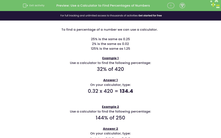# Use a Calculator to Find Percentages of Numbers

In this worksheet, students will use a calculator to find percentages of numbers.Key stage:  KS 3

Curriculum topic:   Number

Curriculum subtopic:   Use Calculators/Technology for Accuracy

Difficulty level:#### Worksheet Overview

To find a percentage of a number we can use a calculator.

However, not all calculators have a % button, so we need to know what to tap into the calculator in order to find our answer!

The easiest method is to find a multiplier. We do this by dividing our percentage by 100.

25% is the same as 0.25

2% is the same as 0.02

125% is the same as 1.25

Did you spot that all those percentages above had been divided by 100 to get the decimal to use as our multiplier?Example 1

Use a calculator to find the following percentage:

32% of 420

First, find our multiplier by dividing the percentage by 100.

32 ÷ 100 = 0.32

0.32 x 420 = 134.4

Another way to work it out would be to type:

32 x 420 = 13,440

13,440 ÷ 100 = 134.4

Example 2

Use a calculator to find the following percentage:

144% of 250

Find the multiplier by dividing the percentage by 100.

144 ÷ 100 = 1.44

1.44 x 250 = 360

Or you could type:

144 x 250 = 36,000

36,000 ÷ 100 = 360

As you can see, there is more than one way to find percentages using a calculator. If your calculator has a % button on it, you will be able to just type in the number followed by the percentage sign.

Let's have a go at some questions now.

### What is EdPlace?

We're your National Curriculum aligned online education content provider helping each child succeed in English, maths and science from year 1 to GCSE. With an EdPlace account you’ll be able to track and measure progress, helping each child achieve their best. We build confidence and attainment by personalising each child’s learning at a level that suits them.

Get started